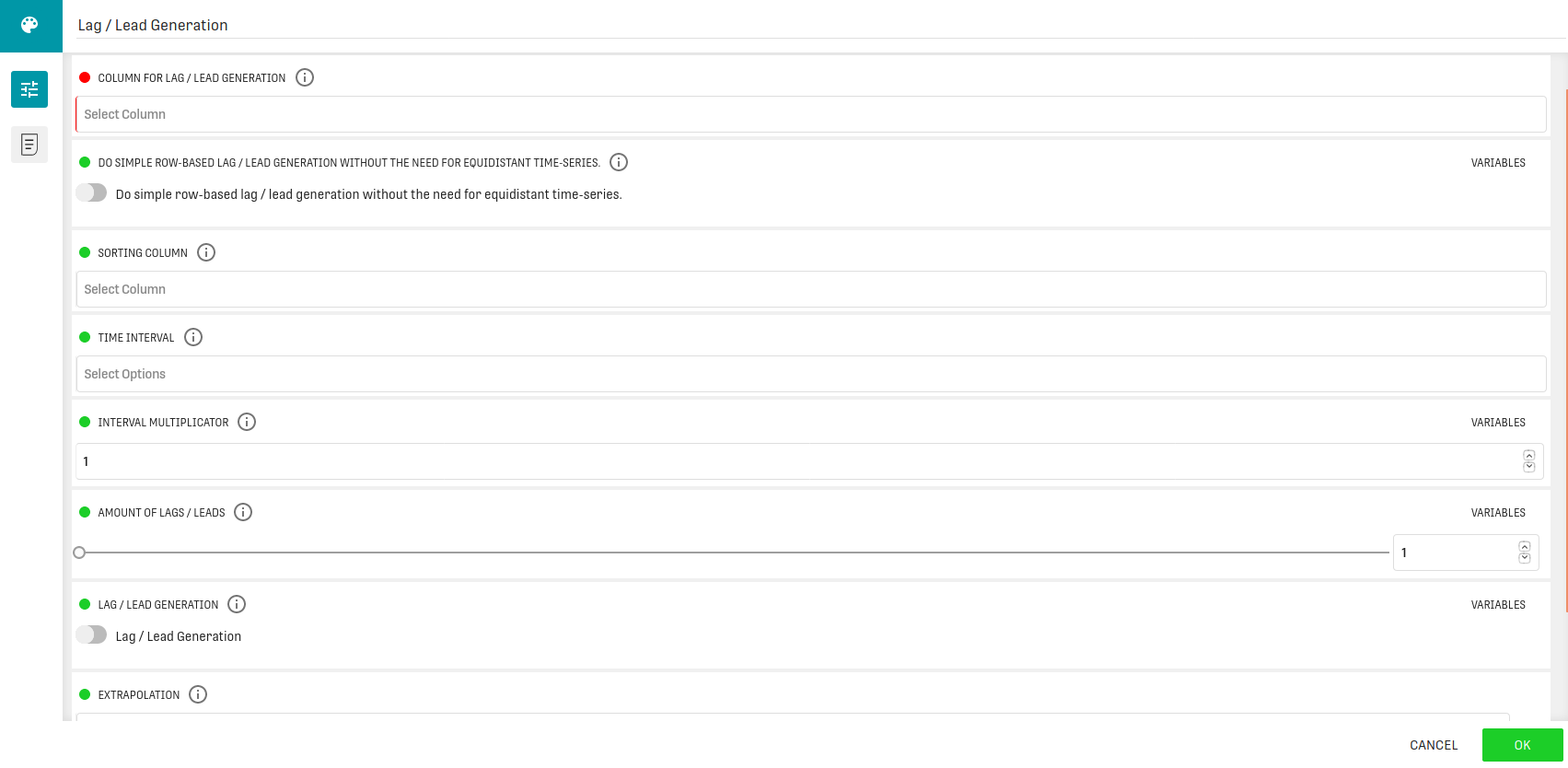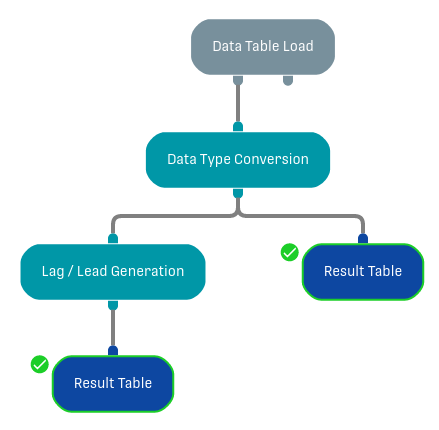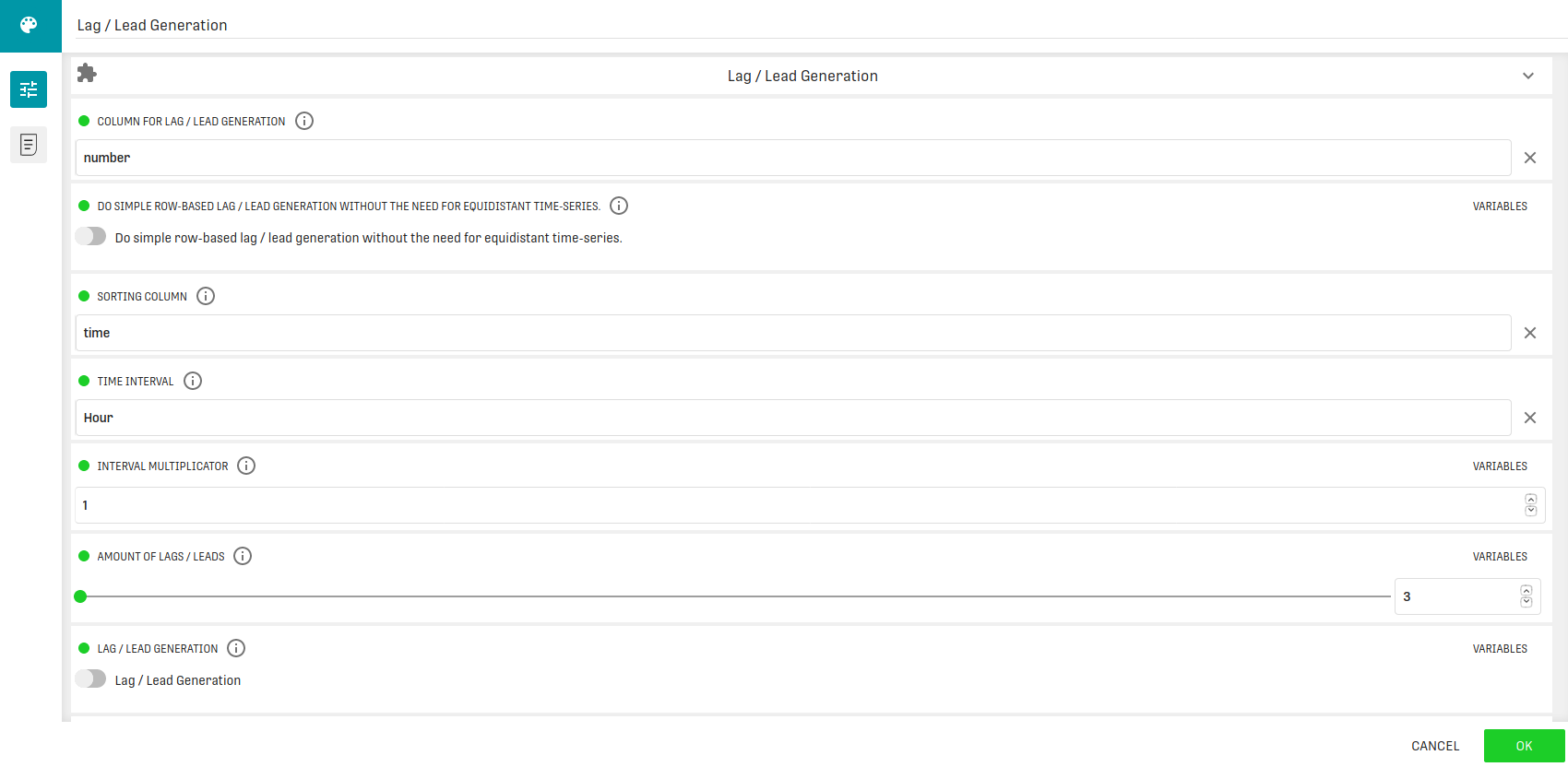Modified on Tue, 30 Nov 2021 at 02:59 PM

# Overview

The Lag Generation Processor creates a lag on a selected column based on a time interval. The generation of lags is often necessary for time series analyses (e.g., ARMA or ARIMA models).

# Input

The processor needs a sequentially ordered timestamp variable and a column containing ratio-scaled observations.

# Configuration### Column For Lag / Lead Generation

Column for which the lag / lead values should be generated. This column may have any type, no restrictions apply here.

### Do Simple Row-Based Lag / Lead Generation Without The Need For Equidistant Time-Series

When set, the column selected in "Sorting column" is only used for sorting the input data before lag / lead generation and does not need to be a datetime column, but can be any sortable column.

If no sorting column is given, we assume the input data is already ordered. Each lag / lead is directly referring to its preceding / succeeding row(s).

### Sorting Column

This configuration option can be used in three different ways:

• If time-based lag / lead generation is done (No row-based lag / lead generation, equidistance of time-stamps is mandatory), the chosen column needs to contain values of type datetime.
• If row-based lag / lead generation is done (check the row-based lag / lead generation option) this option may be not set, then we assume the incoming data is sorted.
• The option is set to a column that is used for sorting the dataset before applying the lag / lead generation (doesn't need to be a datetime column, but can be any column with scale type interval or ratio).

### Time Interval

The time interval which should be used for lag / lead generation, if it is not row-based. This interval can be further customized by choosing a multiplicator in the next config option.

### Interval Multiplicator

When using time-based lag / lead generation, the chosen interval can be further customized (E.g. by using the interval seconds and the value 2 here, we have a time-lag of 2 seconds). When using row-based lag / lead generation, this option is used as a span between the value and its lagged value, e.g. by setting 2 here and lag generation, the first lag of a row is not from the previous row, but from the row before.

The default value for this option is 1.

### Amount Of Lags / Leads

Define the amount of lags / leads to create.

Switch between lag (toggle is off) or lead (toggle is on) generation:
• lag - values for generated column lags are taken from the previous rows of the dataset and new column names have a “_LAG” suffix,
• lead - values for generated column leads are taken from the next rows of the dataset and new column names have a “_LEAD” suffix.

### Exploration

Which kind of extrapolation should be done:
• Delete edge rows - Only keep rows in the result, for which the lags can be calculated.
• Pad with NULL - Keep the edge rows and set their lags to NULL.
• Fill with first / last value - Keep the edge rows and set their lags to first lag value.

### Columns For Grouping

Column, which is used for grouping the data before creating lags / leads of it. Lag / Lead generation will be done for each group separately. A group is defined as a distinct combination of values present in the selected columns. Selecting a value here implicitly makes it mandatory to select a column in "Sorting column".

# Output

The dataset containing new columns with the leading/lagged observations.

# Example

In this example we want to apply a lag to a dataset.

## Input

As input we use a small sample dataset (24 rows) that contains a timestamp, a character and a corresponding number in each row. Here is a snippet of it:

 time character number 00:00 a 1 01:00 b 2 02:00 c 3 03:00 d 4 04:00 e 5

The whole dataset is attached at the the end of the article.

## Workflow

In this workflow we use a Data Table Load Processor to load the dataset, then we convert the strings in the "Time" Column to actual datetime values with a Data Type Conversion Processor. The output of the conversion is passed to a Result Table and to the Lag / Lead Processor, which creates the lag and then saves it again to a Result Table.## ConfigurationThe example configuration has the following settings:

• Column For Lag / Lead Generation: number
• Sorting Column: time
• Time Interval: "Hour"
• Amount of Lags / Leads: 3

For the remaining options the default value is used.

## Result